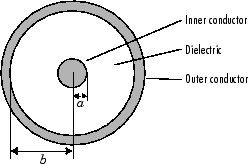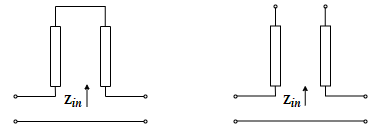Coaxial Transmission Line

Model coaxial transmission line

• Library:
• RF Blockset / Equivalent Baseband / Transmission Lines

•Description

The Coaxial Transmission Line block uses S-parameters to model its frequency behavior. A cross-section of the Coaxial Transmission Line is shown in the following figure. Its physical characteristics include the radius of the inner conductor a and the radius of the outer conductor b.Parameters

expand all

Main

Radius of the outer conductor of the coaxial transmission line, specified as a scalar in meters.

Radius of the inner conductor of the coaxial transmission line, specified as a scalar in meters.

Relative permeability of the dielectric, expressed as the ratio of the permeability of the dielectric to permeability in free space μ0, specified as a real scalar.

Relative permittivity of the dielectric, expressed as the ratio of the permittivity of the dielectric to permittivity in free space ε0, specified as a real scalar.

Loss tangent of the dielectric, specified as a nonnegative scalar.

Conductivity of the conductor, specified as a positive scalar in Siemens/meters.

Physical length of the transmission line, specified as a positive scalar in meters.

The block enables you to model the transmission line as a stub or as a stubless line.

Stubless Transmission Line

• Not a stubNot a stub

If you model a coaxial transmission line as stubless line, the Coaxial Transmission Line block first calculates the ABCD-parameters at each frequency contained in the modeling frequencies vector. It then uses the abcd2s function to convert the ABCD-parameters to S-parameters. For more information, see Stub Mode - Not a Stub.

Shunt Transmission Line

• Shunt—This parameter provides a two-port network that consists of a stub transmission line that you can terminate with either a short circuit or an open circuit as shown in these diagrams.Zin is the input impedance of the shunt circuit and it is calculated as follows:

for a short circuited transmission line

${\text{Z}}_{\text{in}}=j{Z}_{0}\mathrm{tan}\gamma l$

and for the open circuited transmission line

${\text{Z}}_{\text{in}}=-j{Z}_{0}\mathrm{cot}\gamma l$

where

• Z0 is the characteristic impedance and

• $\gamma$ is the propagation constant

The ABCD-parameters for the shunt stub are calculated as

$\begin{array}{c}A=1\\ B=0\\ C=1/{Z}_{in}\\ D=1\end{array}$

Series Transmission Line

• Series—This mode parameter provides a two-port network that consists of a series transmission line that you can terminate with either a short circuit or an open circuit as show in these diagrams.Zin is the input impedance of the series circuit. The ABCD-parameters for the series stub are calculated as

$\begin{array}{c}A=1\\ B={Z}_{in}\\ C=0\\ D=1\end{array}$

Stub termination for stub modes Shunt and Series. Choices are Open or Short

Dependencies

To enable this parameter, select Shunt or Series in Stub mode

Visualization

Frequency data source, specified as User-specified.

Frequency data range, specified as a vector in hertz.

Reference impedance, specified as a nonnegative scalar in ohms.

Type of data plot to visualize using the given data, specified as one of the following:

• X-Y plane — Generate a Cartesian plot of the data versus frequency. To create linear, semilog, or log-log plots, set the Y-axis scale and X-axis scale accordingly.

• Composite data — Plot the composite data. For more information, see Create Plots.

• Polar plane — Generate a polar plot of the data. The block plots only the range of data corresponding to the specified frequencies.

• Z smith chart, Y smith chart, and ZY smith chart — Generate a Smith® chart. The block plots only the range of data corresponding to the specified frequencies.

Type of parameters to plot, specified as one of the following.

 S11 S12 S21 S22 GroupDelay GammaIn GammaOut VSWRIn VSWROut OIP3 IIP3 NF NFactor NTemp TF1 TF2 TF3 Gt Ga Gp Gmag Gmsg GammaMS GammaML K Delta Mu MuPrime

Note

Y parameter1 is disabled when you select Plot type to Composite data.

Type of parameters to plot, specified as one of the following.

 S11 S12 S21 S22 GroupDelay GammaIn GammaOut VSWRIn VSWROut OIP3 IIP3 NF NFactor NTemp TF1 TF2 TF3 Gt Ga Gp Gmag Gmsg GammaMS GammaML K Delta Mu MuPrime

Note

Y parameter2 is disabled when you select Plot type to Composite data.

Plot format, specified as one of the following.

Y parameter1Y format1
S11, S12, S21, S22, GammaIn, GammaOut, TF1, TF2, TF3, GammaMS, GammaML, and Delta.dB, Magnitude (decibels), Abs, Mag, Magnitude (linear), Angle, Angle(degrees), Angle(radians), Real, Imag, and Imaginary.
GroupDelayns, us, ms, s, and ps.
VSWRIn and VSWROut.Magnitude (decibels) and None.
OIP3 and IIP3.dBm, W, and mW.
NF dB and Magnitude (decibels).
NFactor, K, Mu, and MuPrime.None
NTempKelvin
Gt, Ga, Gp, Gmag, and Gmsg.dB, Magnitude (decibels), and None.

Dependencies

To enable Y format1, set Plot type to X-Y plane.

Plot format, specified as one of the following.

Y parameter2Y format2
S11, S12, S21, S22, GammaIn, GammaOut, TF1, TF2, TF3, GammaMS, GammaML, and Delta.dB, Magnitude (decibels), Abs, Mag, Magnitude (linear), Angle, Angle(degrees), Angle(radians), Real, Imag, and Imaginary.
GroupDelayns, us, ms, s, and ps.
VSWRIn and VSWROut.Magnitude (decibels) and None.
OIP3 and IIP3.dBm, W, and mW.
NF dB and Magnitude (decibels).
NFactor, K, Mu, and MuPrime.None
NTempKelvin
Gt, Ga, Gp, Gmag, and Gmsg.dB, Magnitude (decibels), and None.

Dependencies

To enable Y format2, set Plot type to X-Y plane.

Frequency plot, specified as Freq.

Frequency plot format, specified as one of the following.

 Auto Hz kHz MHz GHz THz

Y-axis scale, specified as Linear or Log.

Dependencies

To enable this parameter, set Plot type to X-Y plane.

X-axis scale, specified as Linear or Log.

Dependencies

To enable this parameter, set Plot type to X-Y plane.

Plot the specified data using the plot button.